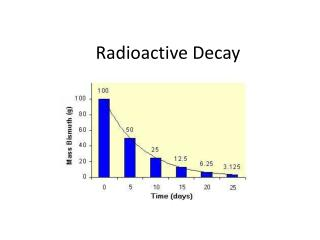DownloadDownload PresentationTélécharger la présentation- - - - - - - - - - - - - - - - - - - - - - - - - - - E N D - - - - - - - - - - - - - - - - - - - - - - - - - - -
##### Presentation Transcript

2. I can solve half-life problems. • Radioisotopes decay in a predictable way • The time is takes for half of a sample to decay is called the half life for the isotope. • Every radioactive element has a unique half-life • C-14 is 5730 years • U-238 is 4.5 billion years • I-131 is 8.05 days

3. I can solve half-life problems • Barium-122 has a half life of 2 minutes. A fresh sample weighing 80 g was obtained. If it takes 10 minutes to set up the experiment using barium-122, how much barium-122 will be left when the experiment begins

4. I can solve half-life problems How many half-lives have passed when 25% of parent isotope remains? What percent of the parent isotope remains after 5 half-lives? If we started with 200 grams of a radioisotope, how much would be left after 2 half-lives?

5. I can solve half – life problems What is the half-life of strontium-90? How do you know? How many half-lives have passed when 12.5 grams of Sr-90 remain? How much Sr-90 remains after 8 half-lives?

6. I can solve half-life problems • Use the formula • The amount remaining is equal to the original amount times 1/2 raised to the # of half-lives that have passed. N=N0(1/2)n • N= amount of remaining radioisotope • N0= original amount of radioisotope sample • n= # of half lives that have passed (time elapsed/half life)

7. I can solve half-life problems • Barium-122 has a half life of 2 minutes. A fresh sample weighing 80 g was obtained. If it takes 10 minutes to set up the experiment using barium-122, how much barium-122 will be left when the experiment begins • N=N0(1/2)n • N=? • N0=80g • n= time elapsed/half life = 10 days/2 days = 5 • N= 80g (1/2)5 • N=80g (1/2)(1/2)(1/2)(1/2)(1/2) • N= 2.5 grams Barium-122

8. I can solve half-life problems • Actinium-226 has a half-life of 29 hours. If 100 mg of actinium-226 decays over a period of 58 hours, how much actinium-226 will remain? • N=N0(1/2)n • N=? • N0=100 mg Ac • n= 58 days/29 days= 2 half lives pass • N= 100 mg (1/2)2 • N=25 g Ac

9. I can solve half-life problems • Iodine-131 is used to destroy thyroid tissue in the treatment of an overactive thyroid. The half-life of iodine-131 is 8 days. If a hospital receives a shipment of 200 g of iodine-131, how much I-131 would remain after 32 days? • N=N0(1/2)n • N=? • N0=200 g I • n= 32 days/8 days= 4 half lives • N= 200g (1/2)4 • N= 12.5 g I

10. I can solve half-life problems • Mercury -197 is used for kidney scans and has a half-life of 3 days. If the amount of mercury-197 needed for a study is 1.0 gram and the time allowed for shipment is 15 days, how much mercury-197 will need to be ordered? • N=1.0 grams Hg-197 • N0= ? • n=15 days/3 days= 5 half lives • 1.0grams= N0(1/2)5 • N0=32 grams